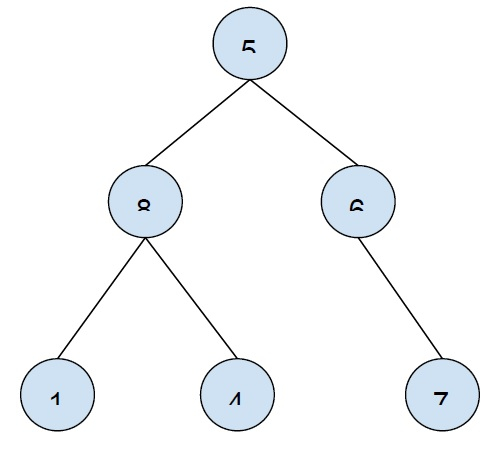# Print All Leaf Nodes of a Binary Tree from left to right using Iterative Approach in C++

In this problem, we are given a binary tree and we have to print all leaf nodes of the binary tree from left to right the iterative approach.

Let’s take an example to understand the problem

Input −Output − 1 4 7

To solve this problem using the iterative approach, we will use a Depth-first search(DFS). To Traverse tree, we will start from root node and check if it is a leaf node if it is then print the node else find its child trees and traverse the child subtrees to find all leaf nodes.

## Example

The below code will implement our solution −

Live Demo

#include <iostream>
using namespace std;
struct Node {
int data;
struct Node *left, *right;
};
Node* insertNode(int data) {
Node *temp = new Node;
temp->data = data;
temp->left = temp->right = NULL;
return temp;
}
void printLTRLeafNodes(Node *root){
if (!root)
return;
if (!root->left && !root->right) {
cout<<root->data<<"\t";
return;
}
if (root->left)
printLTRLeafNodes(root->left);
if (root->right)
printLTRLeafNodes(root->right);
}
int main(){
Node *root = insertNode(21);
root->left = insertNode(5);
root->right = insertNode(36);
root->left->left = insertNode(2);
root->right->left = insertNode(13);
root->right->right = insertNode(4);
root->right->left->left = insertNode(76);
root->right->left->right = insertNode(9);
root->right->right->left = insertNode(17);
root->right->right->right = insertNode(2);
cout<<"Leaf Nodes of the tree from left to rigth are :\n";
printLTRLeafNodes(root);
return 0;
}

## Output

Leaf Nodes of the tree from left to right are −
2 76 9 17 2